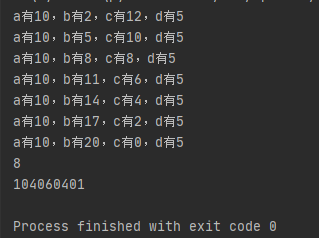2021-05-26 11:11

一道python题目

• 写回答
• 好问题 提建议
• 追加酬金
• 关注问题
• 收藏
• 邀请回答

4条回答默认 最新

•CSDN专家-黄老师 2021-05-26 11:23
已采纳

问题1

# 四层循环
num = 1         # 用于计算有多少种方案
count = 0       # 用于计算循环的次数
for a in range(0, 101):
for b in range(0, 101):
for c in range(0, 101):
for d in range(0, 101):
count += 1
if a + b*2 + 3*c + d*10 == 100:
print(f'a有{a}，b有{b}，c有{c}，d有{d}')
num += 1
print(num)
print(count)

问题2

# 四层循环
num = 1         # 用于计算有多少种方案
count = 0       # 用于计算循环的次数
for a in range(0, 101):
for b in range(0, 101):
for c in range(0, 101):
for d in range(0, 101):
count += 1
if a + b*2 + 3*c + d*10 == 100 and a==10 and d==5:
print(f'a有{a}，b有{b}，c有{c}，d有{d}')
num += 1
print(num)
print(count)如有帮助，望采纳，谢谢

评论
解决 1 无用
打赏 举报